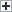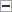Select PageDepreciation is an accounting process where the cost of an asset is accounted for and expensed over its useful life. It shows how the value of the asset decreases over time. Assets that can be depreciated include buildings, fixtures, production equipment, etc. For intangible assets, including many types of intellectual property, this process is called amortization. For commodities mined or harvested from the earth, such as lumber, crude oil or natural gas, this process is called depletion. Here are four common types of depreciation.

Straight Line Method

In order to determine depreciation using this method, the following formula is used:

Depreciation = (Asset cost – Salvage value) / Useful life

The salvage value is the asset’s remaining value after its useful life, and the remaining amount from the asset’s cost is depreciable. The depreciable amount is divided by the asset’s useful life that’s used for depreciation expensing.

Double Declining Balance Depreciation

In order to calculate this method of depreciation, the first step is to look at the asset cost. From there, its useful life must be established. Let’s assume an asset’s book value is \$75,000, it has a useful life of 10 years and a salvage value of \$8,050.

Depreciation = (100 percent / asset’s useful life) X 2

= (100 percent / 10) X 2 = 20 percent

Year 1 depreciation expense = \$75,000 X 20 percent = \$15,000

Year 2 depreciation expense = \$60,000 (\$75,000 – \$15,000 from Year 1) X 20 percent = \$12,000

When beginning the first year, the book value is used as a basis for the asset’s value. The ending book value, which is determined after subtracting depreciation, is the following year’s new book value that will be used to establish next year’s depreciation expense. After it’s repeated through its useful life, the salvage value is left.

Units of Production Depreciation Method

This type of depreciation method depreciates a business’ asset by the units it produces or how many hours the asset is to be run for production over its useful life.

Depreciation = (Number of items manufactured / useful life in measured units) X (asset cost – salvage value)

Let’s assume a supplement pill machine costs \$50,000; it can produce 200 million vitamins over its lifetime; and it will have a salvage value of \$2,500. This assumes it will produce 20 million vitamins in the first 12 months of operation.

(20 million / 200 million) = 10 percent X (\$50,000 – \$2,500) = \$47,500

If the machine produces 10 percent of vitamins over its expected 200 million vitamin unit life, the resulting depreciation amount is \$4,750. At the end of the first year the book value will be \$45,250. Production amounts in future years will dictate how much may be depreciated.

Sum of the Years Digits Approach

Similar to other methods of depreciation, the Sum of the Years Digits (SYD) depreciation method is another type of depreciation that assigns the bulk of depreciation in the beginning years of an asset’s useful life. Looking at the formula is the best way to understand how it works.

Expensing Depreciation = (Asset’s remaining life / Sum of the years digits) X (Asset’s cost – salvage value)

If a machine that’s going to be used by a company to produce widgets costs \$50,000, has a useful life of 16 years and a salvage value of \$3,000, it would look as follows:

1. \$50,000 – \$3,000 = \$47,000 Depreciation Base

2. With 16 years of useful life for the asset, the sum of the years would be: 1 + 2 + 3 + 4 + 5 +6 + 7 + 8 + 9 + 10 + 11 + 12 + 13 + 14 + 15 + 16 = 136. Using the machine referenced above during the first year would equal a Remaining Life of 16. Then, the Remaining Life of 16 years would be divided by the SYD of 136.

3. Using this example, for the first year of using the machine, the formula would be as follows:

16 years (remaining life) / 136 (SYD) = 0.11764. Then, 0.11764 X \$47,000 (Depreciation Base) = \$5,529.08

The next (or second) year’s depreciation expense would by 15 / 136 = 0.110294. Then, 0.110294 X \$47,000 = \$5,183.82

Each subsequent year the SYD would be divided by the remaining years until it’s exhausted and the salvage value should be met.

Depending on the type of business, the type of asset and the accounting approach, there are different ways to expense for property acquired during the course of business.

Disclaimer